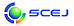English page## \$B^5-G09V1i(B

\$B:G=*99?7F|;~!'(B2015-03-05 09:42:37

### \$B3X2q>^(B

 \$BBh(B 1 \$BF|!&(BZ \$B2q>l(B Z104 \$B!V(B(\$B8&5fBjL\(B)\$BHyN3;R%\%H%`%"%C%W%W%m%;%9\$NAO@.\$K4X\$9\$k8&5f!W(B (10:00\$B!A(B10:30) (\$BElKLBg(B) \$B:#Ln(B \$B44CK(B \$B;a(B Z105 \$B!V(B(\$B8&5fBjL\(B)\$B%<%*%i%\$%HKl\$r\$O\$8\$a\$H\$9\$k%J%NB?9& (10:30\$B!A(B11:00) (\$B;38}Bg(B) \$B4nB?(B \$B1QIR(B \$B;a(B

### \$B8&5f>^(B

 \$BBh(B 3 \$BF|!&(BB \$B2q>l(B B320 \$B!V(B(\$B8&5fBjL\(B)\$B6I=jE*N.F0@)8f5Z\$S@EEE>l\$rMxMQ\$7\$?9b@:EYHyN3;RJ,5i\$K4X\$9\$k8&5f!W(B (15:20\$B!A(B15:40) (\$B9-Bg(B) \$B5HED(B \$B1Q?M(B \$B;a(B

### \$B8&5f>)Ne>^(B

 \$BBh(B 3 \$BF|!&(BC \$B2q>l(B C309 \$B!V(B(\$B8&5fBjL\(B)\$BGvKl43>DN.F02hA|K!\$rMQ\$\$\$?9bJ,;R?-D9\$,MpN.M^@)\$KM?\$(\$k1F6A\$N2rL@!W(B (11:40\$B!A(B12:00) (\$B?@8MBg(B) \$BF|=P4V(B \$B\$k\$j(B \$B;a(B \$BBh(B 3 \$BF|!&(BE \$B2q>l(B E309 \$B!V(B(\$B8&5fBjL\(B)\$B%P%\$%*%^%F%j%"%k\$H\$7\$F\$N9)3XE*1~MQE83+\$rL\;X\$7\$??75,5!G=@-%R%I%m%2%k\$NAO@=!W(B (11:40\$B!A(B12:00) (\$B \$BBh(B 3 \$BF|!&(BK \$B2q>l(B K313 \$B!V(B(\$B8&5fBjL\(B)\$B2=3X%W%i%s%H\$K\$*\$1\$k@)8f@-G=8~>e\$N\$?\$a\$N?dDj@)8f (13:00\$B!A(B13:20) (\$BElBg(B) \$B6b;R(B \$B90>;(B \$B;a(B

### \$B5;=Q>^(B

 \$BBh(B 1 \$BF|!&(BE \$B2q>l(B E118 \$B!V(BDEM\$B\$K\$h\$k?77?J4BN:.9g5!\$N3+H/\$H\$=\$N@=IJ2=!W(B (14:40\$B!A(B15:00) (\$BFA\$B!&(B \$B5HED(B \$BBY;0(B \$B;a(B\$B!&(B \$BD+F|(B \$B@5;0(B \$B;a(B\$B!&(B \$BKY9g(B \$B@?(B \$B;a(B E119 \$B!V(BJPE\$B4%<0C&N2C&>K%W%m%;%9(B(ReACT:Regenerative Activated Coke Technology)\$B!W(B (15:00\$B!A(B15:20) (\$B%8%'%\$%Q%o!\$B!&(B \$BGr0f(B \$B900l(B \$B;a(B\$B!&(B \$B?@C+(B \$B0l7{(B \$B;a(B\$B!&(B \$B>.DH(B \$B9,0l(B \$B;a(B\$B!&(B \$B5\(B \$B@59@(B \$B;a(B

### \$B%"%8%"9q:]>^(B

 \$BBh(B 2 \$BF|!&(BG \$B2q>l(B G203 \$B!V(BHigh value utilization and efficient transformation mechanisms of renewable bioresource\$B!W(B (9:30\$B!A(B10:10) (Beijing U. Chemical Tech.) Su Haijia \$B;a(B \$BBh(B 2 \$BF|!&(BH \$B2q>l(B H207 \$B!V(BSynthesis and Characterization of Hierarchically Porous Carbons and Composites for Supercapacitors\$B!W(B (11:00\$B!A(B11:40) (Nat. Tsing Hua U.) Hu Chi-Chang \$B;a(B

### \$B=w@->^(B

 \$BBh(B 2 \$BF|!&(BN \$B2q>l(B N210 \$B!V9)6H2=%W%m%8%'%/%H\$NBi8oL#!";R0i\$F\$bA3\$j!W(B (12:10\$B!A(B12:30) (\$B%+%M%+(B) \$B5H8+(B \$BCNJf(B \$B;a(B N211 \$B!V\$b\$&\$R\$H>!Ii!W(B (12:30\$B!A(B12:50) (\$BEl9)Bg(B) \$BLnB<(B \$B=_;R(B \$B;a(B

\$B9V1iH/I=%W%m%0%i%`(B
\$B2=3X9)3X2q(B \$BBh(B80\$BG/2q(B(C) 2015 \$B8x1W
Most recent update: 2015-03-05 09:42:37
E-mail: inquiry-80awww3.scej.org
This page was generated byeasp 2.36; proghtml 2.37 (C)1999-2015 kawase